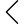880 products found
• s
• m
• l
• xl
• s
• m
• l
• xl
• s
• m
• l
• xl
• s
• m
• l
• xl
• s
• m
• l
• xl
• s
• m
• l
• xl
• s
• m
• l
• xl
• s
• m
• l
• xl
• s
• m
• l
• xl
• s
• m
• l
• xl
• s
• m
• l
• xl
• s
• m
• l
• xl
• s
• m
• l
• xl
• s
• m
• l
• xl
• 2xl
• s
• m
• l
• xl
• s
• m
• l
• xl
• s
• m
• l
• xl
• s
• m
• l
• xl
• s
• m
• l
• xl
• 2xl
• 3xl
• s
• m
• l
• xl
• s
• m
• l
• xl
• s
• m
• l
• xl
• s
• m
• l
• xl
• s
• m
• l
• xl
• s
• m
• l
• xl
• s
• m
• l
• xl
• s
• m
• l
• xl
• s
• m
• l
• xl
• s
• m
• l
• xl
• xxl
• s
• m
• l
• xl
• s
• m
• l
• xl
• s
• m
• l
• xl
• s
• m
• l
• xl
• s
• m
• l
• xl
• s
• m
• l
• xl
• s
• m
• l
• xl
• s
• m
• l
• xl
• s
• m
• l
• xl
•• 1
• 2
• 3
• 22